Courses

# Short Notes - Force and Laws of Motion Class 9 Notes | EduRev

## Class 9 : Short Notes - Force and Laws of Motion Class 9 Notes | EduRev

The document Short Notes - Force and Laws of Motion Class 9 Notes | EduRev is a part of the Class 9 Course Class 9 Science by VP Classes.
All you need of Class 9 at this link: Class 9

Facts that Matter

• Force: It is a push or pull on an object that produces acceleration in the body on which it acts. S.I. unit of force is Newton.
• A force can do three things on a body.
(a)It can change the speed of a body.
(b)It can change the direction of motion of a body.
(c)It can change the shape and size of a body.
• Balanced forces: Forces are said to be balanced forces if they nullify one another and their resultant force is zero.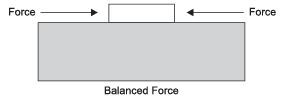• Unbalanced forces: When two opposite forces acting on a body, move a body in the direction of the greater force or forces which brings motion in a body are called as unbalanced forces.
•  First law of motion: An object remains in a state of rest or of uniform motion in a straight line unless acted upon by an external unbalanced force.
•  Inertia: The natural tendency of an object to resist a change in its state of rest or of uniform motion is called inertia.
The mass of an object is a measure of its inertia.
Its S.I. unit is kg.
A body with greater mass has greater inertia.
• Frictional force: The force that always opposes the motion of objects is called force of friction.
• Second law of motion: The rate of change of momentum of an object is proportional to the applied unbalanced force in the direction of the force.

Mathematically,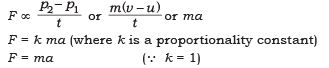• Momentum: The momentum of an object is the product of its mass and velocity and has the same direction as that of the velocity. Its S.I. unit is kg m/s.
(p = mv )

1 Newton: A force of one Newton produces an acceleration of 1 m/s2 on an object of mass 1kg.
1N = 1 kg m/s2
(F = ma)

• Third law of motion: To every action, there is an equal and opposite reaction and they act on two different bodies.
• Conservation of momentum: If the external force on a system is zero, the momentum of the system remains constant i.e., in an isolated system, the total momentum remains conserved.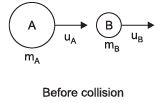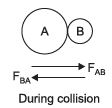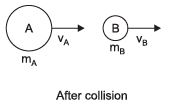Suppose A and B are two balls, they have mass mA and mB and initial velocities uA and uB as shown in above figure before collision.

The two bodies collide and force is exerted by each body. There is change in their velocities due to collision. (mAuA + mBuB) is the total momentum of the two balls A and B before collision and (mAv+ mBvB) is their total momentum after the collision.
The sum of momenta of the two objects before collision is equal to the sum of momentum after the collision, provided there is no external unbalanced force acting on them.
This is known as the law of conservation of momentum.

Offer running on EduRev: Apply code STAYHOME200 to get INR 200 off on our premium plan EduRev Infinity!

75 docs|14 tests

,

,

,

,

,

,

,

,

,

,

,

,

,

,

,

,

,

,

,

,

,

;